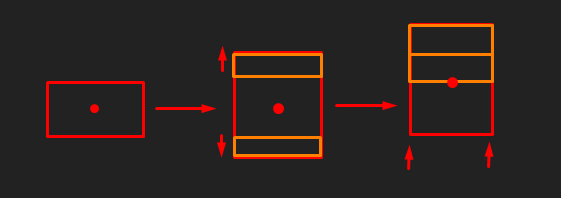# How to scale a part on side through script

Trying to make it where overtime a part will extend on one side. Here is the script that I have.

``````local Lava = game.workspace.lava

local function Move()
while true do
Lava.Size = Lava.Size + Vector3.new(0,0.07,0)
wait()
end
end
``````

Instead of extending the part on one side, it extends both sides of the part on the Y axis. I want it too only extend the part on one side on the Y axis. Thank you.

When increasing the size of a part, the position remains the same. This means that if you make it grow in size vertically, it will add length to the top and bottom since the part itself will not move (if its anchored).Therefore, to make it only extend in one direction, you need to adjust the position by half the increased size.

``````function increaseHeight(part, amount)
part.Size += Vector3.new(0,amount,0)
part.CFrame *= CFrame.new(0,amount/2,0)
end
``````

This function will increase the part’s height by `amount`, and then move the part on its local Y axis in that direction. Just make sure the part is facing the right way.

1 Like

just move the part by (amnt of size it grows by /2)

Okay thanks. So something like this?

``````local Lava = game.workspace.lava

local function increaseHeight(part, amount)
while true do
Lava.Size += Vector3.new(0,amount,0)
Lava.CFrame *= CFrame.new(0,amount/2,0)0)
wait()
end
end
``````

Not quite but you’re getting there;
typically you don’t put a `while true do` inside the function, but instead call the function from somewhere else.
See this example:

``````local Lava = game.workspace.lava

local function increaseHeight(part, amount)
part.Size += Vector3.new(0,amount,0)
part.CFrame *= CFrame.new(0,amount/2,0)
end

while true do
local w = wait()
increaseHeight(Lava, w)
end
``````

Note I put ‘w’ as the amount value, this makes makes it so that if the game stutters or slows down, the part will still go up by the expected speed. To make it faster, simply multiply `w` by some numeric value. Ie: `increaseHeight(Lava, w*10)`

Edit: format

3 Likes